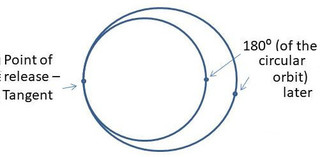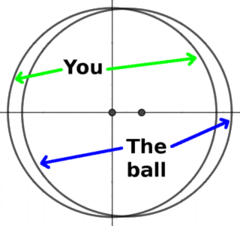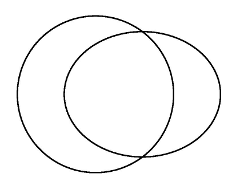# The Fore Aft TideSubscribe!My latest posts can be found here:
Previous blog posts:

## The "Fore-Aft" Tide

In our last post we saw that when we're in orbit, if we throw a ball very gently in exactly the right direction, somehow it bounces back and forth through our position. We experience a fictitious force, pulling the ball back to a resting position, so it moves in Simple Harmonic Motion.

So remember the setup. You're in orbit around the Earth with the International Space Station, and you have a juggling ball. (Don't ask). You hold it next to your helmet and release it, and it just stays there, apparently floating.

Now gently push the ball ahead of you in orbit. In effect you are throwing the ball away from you, and once again, the expectation is that it will drift away from you in a straight line at a constant speed. And once again, it doesn't.

## So what happens this time?

Well, it depends.Exactly tangential
If the throw of the ball is exactly tangentially to your current path then you've added a little energy to its orbit. It then has a slightly elliptical orbit, tangent with yours, and so it takes a little longer to return to its starting point. You initially see it going away from you, then it apparently rises a little, and seems to slow down. You overtake on the underside - you might think of it as undertaking(!) - and it falls behind you. This is the first stage of a Hohmann transfer orbit to an orbit that is microscopically higher - it would normally be matched by an exactly identical "burn" at it's apogee, the highest point in its orbit.

So if we throw the ball exactly tangentially, that's what happens. The ball ends up in an elliptical orbit, but it's a slightly slower orbit.

So let's tweak our direction slightly, let's add a tiny "upwards" component. The throw of the ball is not exactly tangential, but it does end up in an elliptical orbit. More, we can arrange this angle so that the period of that orbit is identical to ours. If the throw is gentle enough the angle to our path is tiny. What happens then?

Remember, we're assuming that we've injected it into an orbit that has the same period as ours, 92 minutes.

This time the ball starts off travelling away from you, but again, it apparently slows down, and again, it comes to a complete halt in 23 (or so) minutes. Then it starts to come back. It passes you (or you overtake it) and it starts to fall behind. As last time it again slows down, comes to a complete stop, and returns. Because the orbital periods match, a complete cycle takes about 92 minutes, and if you have indeed got the direction exactly right (and that's inhumanly accurate, I admin) the ball will gently oscillate through your position, completing a cycle every 92 (or so) minutes. Again, Simple Harmonic Motion.

But what's happening this time?The orbits after the push
This time instead of changing the orientation of the orbit, we're keeping it in the same plane, but changing it from a (conceptually) perfect circle to an ever-so-slightly non-circle ... an ellipse. The diagram at left shows the idea, two orbits that are very nearly the same shape and that stay close together the entire time. But the orbital times match, so they stay "in sync".Exaggerated for clarity
The diagram at right shows the same configuration, but with the eccentricity of the ellipse greatly exaggerated to show the effect.

Now we use the fact that the orbital period is determined only by the length of the semi-major-axis, so provided the ellipse is as wide as the circle, the orbital period will be identical.

Remember, we're assuming that our gentle "push" of the juggling ball hasn't altered its orbital period, so that means the "width" of the ellipse that it now has as its orbit must be same as that of the circular orbit. That, in turn, means that some of the ellipse will be outside the circle, and some inside. The result is that the paths will cross twice. In one case the ball will depart forwards, and in the other we will overtake the ball.

## Another way to understand it

Can we understand this in simple, local dynamics? Yes.

By pushing the ball ahead of us we are giving it a little more speed, so it will rise into a slightly higher orbit. But now it doesn't have enough speed to stay at that greater altitude, and while it initially gets away, the higher orbit is a slower orbit, so we catch up. Then it falls to a lower, slightly faster orbit, catches up with us, and so the cycle repeats. This also requires that our "push" is not exactly tangential, otherwise we get the Hohmann Transfer Orbit as mentioned above. Tricky this, isn't it!

From the outside looking in it's a simple case of slightly different, overlapping orbits with the same orbital period. But from our point of view it's as if there is a mysterious force pulling the ball back to us, and it bounces back-n-forth in Simple Harmonic Motion.

## Quantifying the force

So how big is this mysterious force? Since the period of bounce is the same as the orbital period, exactly the same calculation works as last time, and we get exactly the same equation for the force.

So in each direction, sideways and fore-aft, we have an inwards acceleration of about $1.3x10^{-6} ms^{-2}$ for every metre of displacement. Again, not a lot, but enough to have to know about in some cases.

## Comparing with gravity

Some people wonder if the Simple Harmonic Motion is caused by the gravitational attraction of you, or of the Space Station. We can calculate what mass is needed to have the same attractive force at one metre. The formula is Newton's universal law of gravitation:

• $F=\frac{G~M_1~M_2}{R^2}$.

Using $G\approx 6.673 \times 10^{-11} N\cdot m^2kg^2$, to get a force of about $1.3x10^{-6} ms^{-2}$ at a distance of 1 metre we need a mass of about 20,000 kg. Admittedly that's not huge, but remember that gravitational attraction falls off with the square of the distance, whereas this tidal force grows with distance (up to a point). So while the gravitational force from the ISS is relevant when you're very, very close, you have to be so close that you're actually inside, and then all bets are off.

So no, the gravitational attraction of the ISS isn't really relevant.

Whew!

## And next?

In our next post we'll look at the remaining direction - "up" and "down".

## Acknowledgements

My thanks to Neil Walker for doing the initial calculations of gravitational attraction, Ben Ashforth (Twitter) for a correction about the orbital period, and the "exaggerated" diagram, and Bill Mullins (Twitter) for conversations about transfer orbits, etc.

Their contributions made this post much better than it might have been.

 <<<< Prev <<<< The Sideways Tide : >>>> Next >>>> The Up Down Tides ...You can follow me on Mathstodon.

 Of course, you can alsofollow me on twitter:## Send us a comment ...

 You can send us a message here. It doesn't get published, it just sends us an email, and is an easy way to ask any questions, or make any comments, without having to send a separate email. So just fill in the boxes and then

 Your name : Email : Message :

# ContentsSuggest a change ( <-- What does this mean?) / Send me email